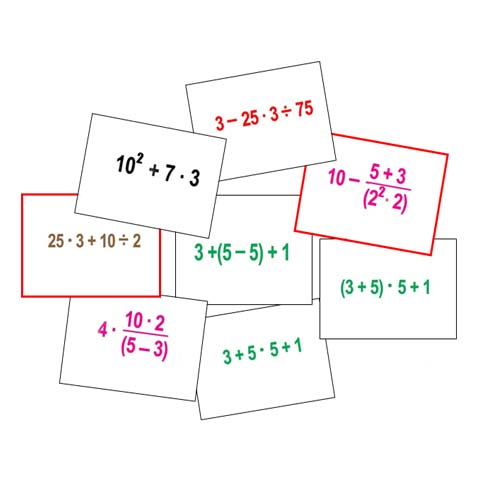STANDARD:  NUMBER SENSE   LEVEL: EM  M - H   PURPOSE: To realize the need for Order of Operations and develop the basic skills of doing the correct order      STRATEGY:                                Parenthesis & Grouping Symbols            Exponents            Multiplying (L to R - either order)            Dividing            Adding (L to R  either order)            Subtracting              DIRECTIONS:  Simplify each expression (find the final answer) by applying the correct Order of Operations. WRITE ANSWERS ON PAPER - promotes a good "math work ethic of using mental math with paper & pencil, as well as, caluclators.   HINT: 1) Recognize that adding & subtracting are done LAST (look at them as terms). For example,               25 x 3 + 10 / 2 do both sides of the addition sign first, then "put together" the two sides. 75 + 5 = 80   2) You must do the top & bottom of the grouping symbols (division line) before you can divide.  For example, before you can subtract something from 10, you have to know what it is.    5+3   = 8 (above the line) (22 x 2) = 8 (below the line) now, divide 8 / 8 = 1 to finish the Grouping Symbols. Now, you have something to subtract from 10. Last, do 10  1 = 9.